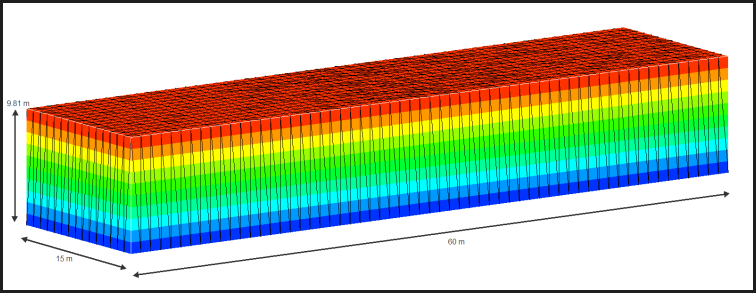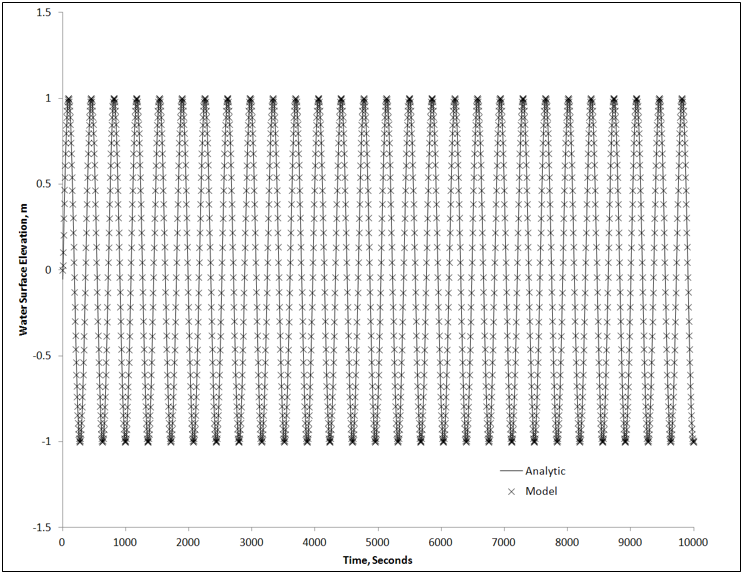## Model Response to Tidal Propagation

This is a test case for the accuracy of the time integration scheme implemented in representing the propagation of an undamped sine wave in a rectangular channel.

Reference

• Taylor, C and Davis, J (1975). "Tidal and Long Wave Propagation", Elsevier, pp 111.

Domain

• Cuboid flume 60 m (length), 15 m (width) and 9.81 m (depth)
• Open at one end, enclosed on all others
• "Free-slip" velocity condition is assumed on all internal wallsInitial Conditions

• Initial depth = 9.81 m

Boundary Conditions

• h = (1.0)Sin(t) ; where h is water surface displacement and t is time

Results

• Taylor and Davis 1975 reports the analytic solution as:
• h = (1.0)Sin(t - x/9.81) ; where x is the longitudinal distance from the open boundary
• Analytic versus model water surface elevation results at X=30 m and Y = 15 m• Error between analytic and model results at X=30 m and Y = 15 m